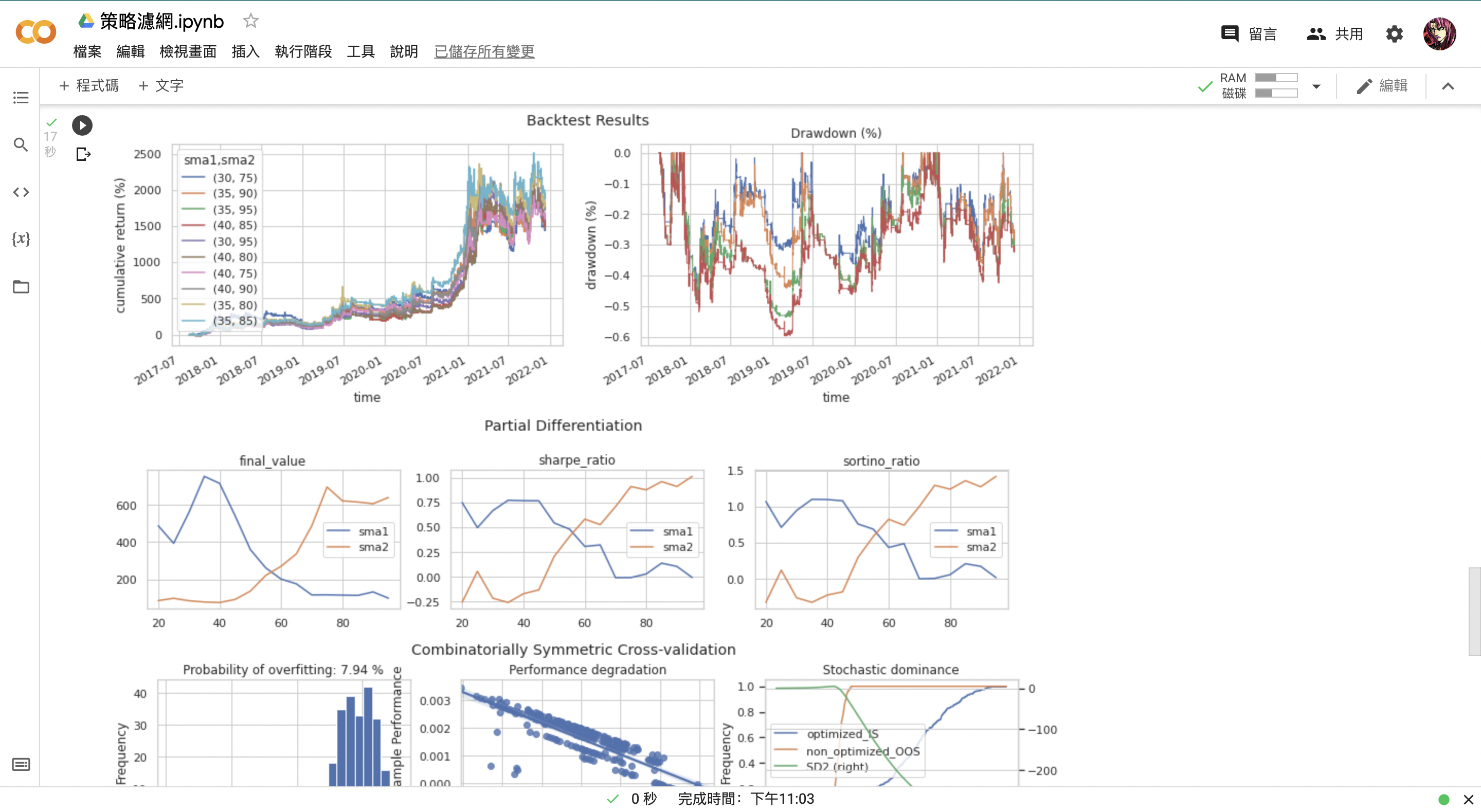# 第二章：4 製作策略濾網

（請參考第一章第一節來啟動該筆記本）

### 1. 什麼是濾網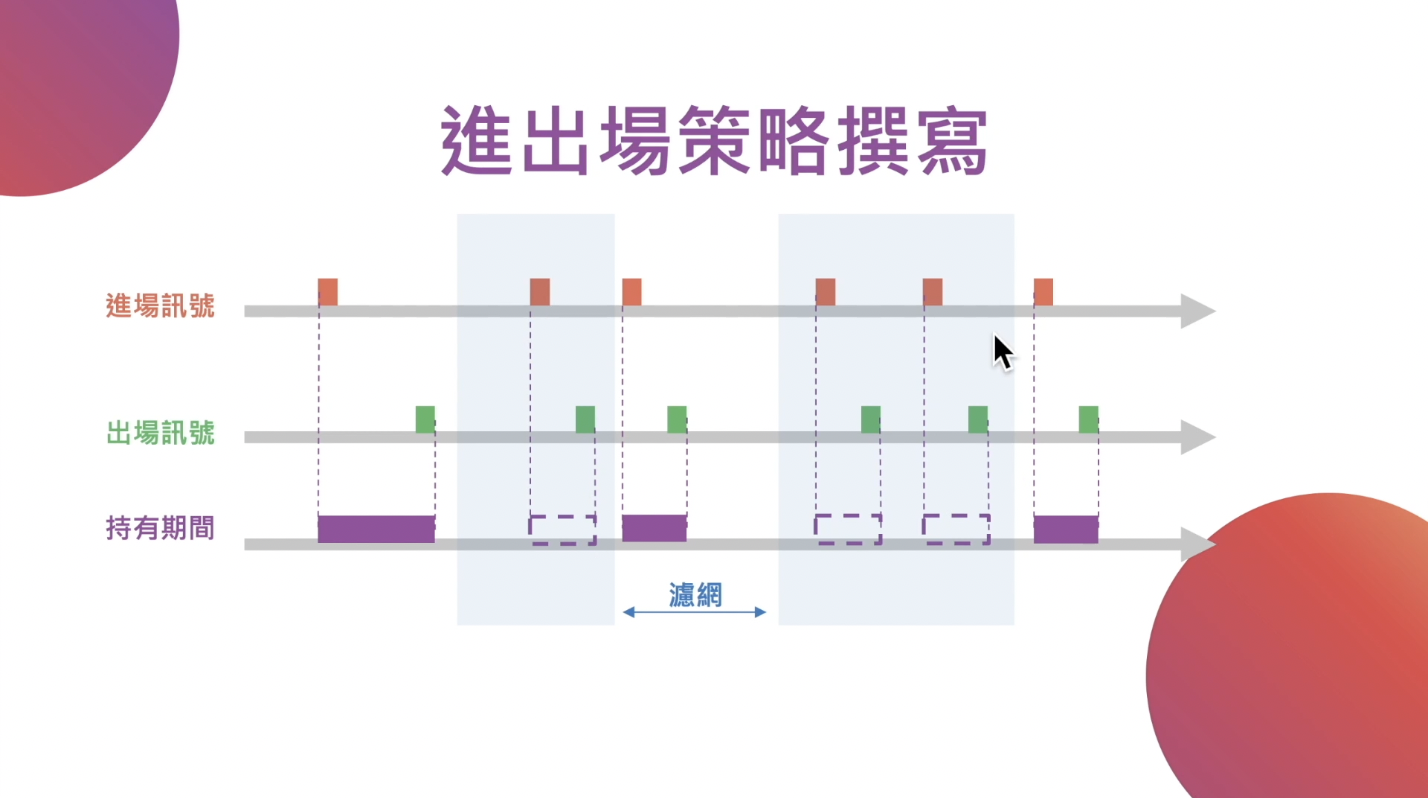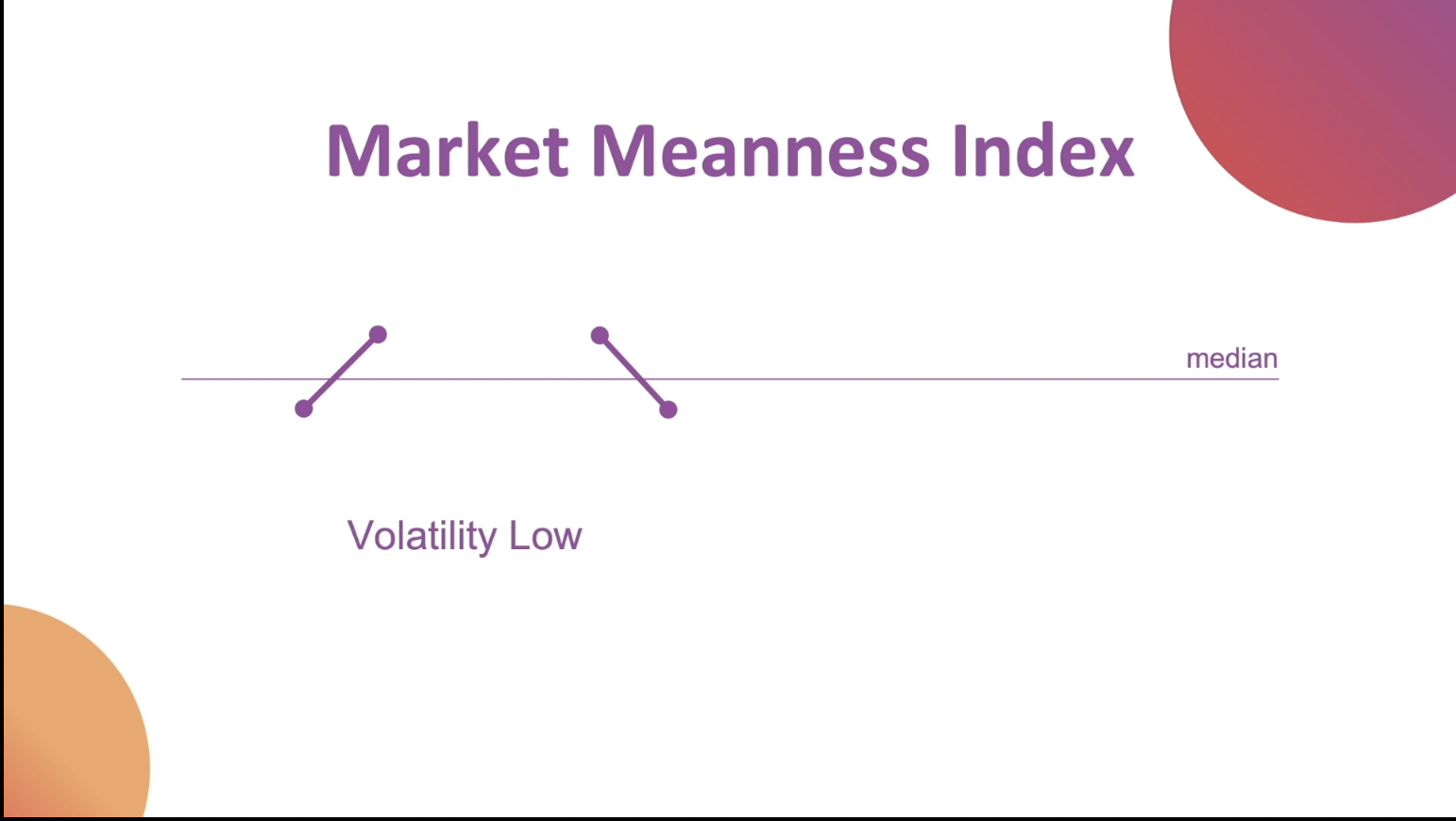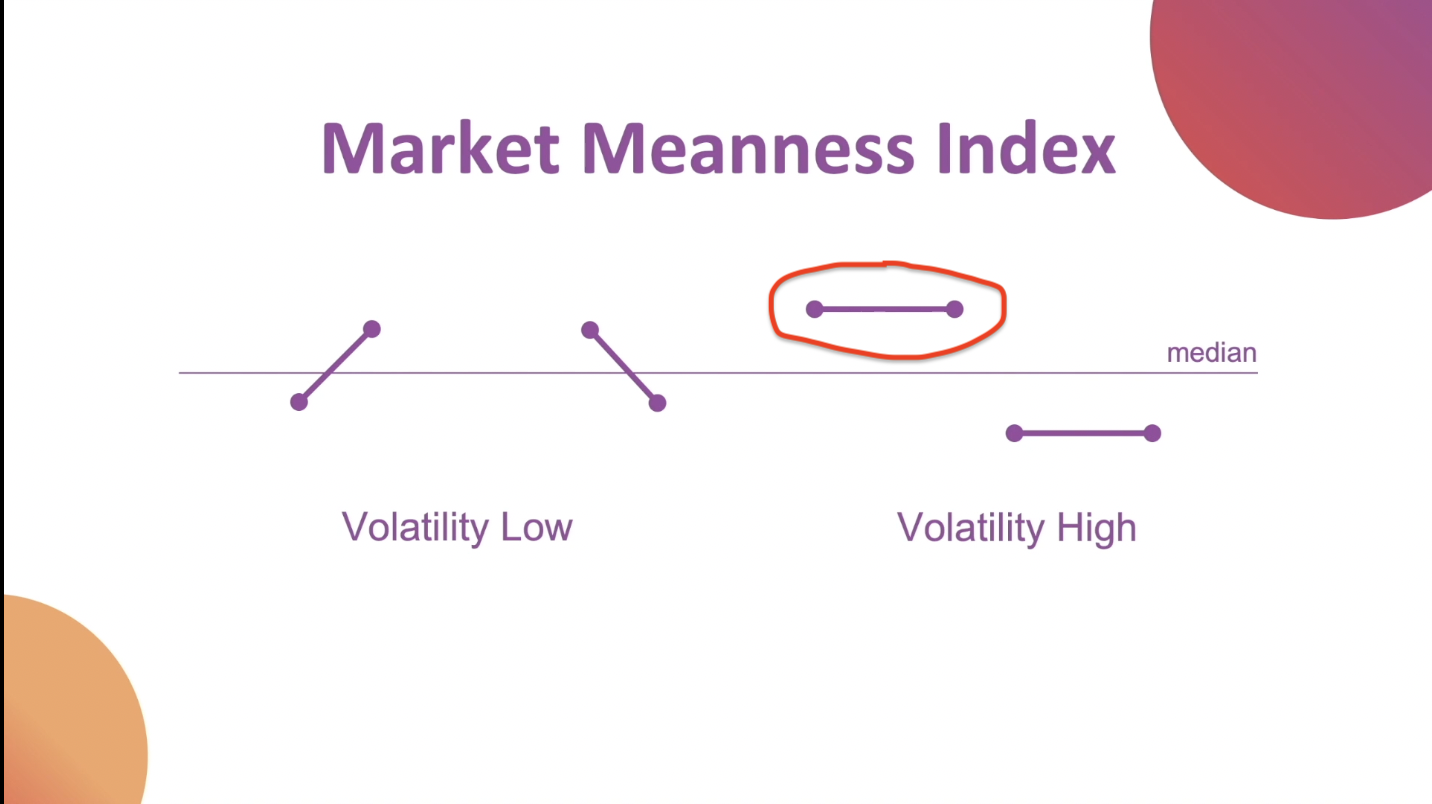### 2. 撰寫策略濾網

median = ohlcv.close.rolling(20).median()


ohlcv.close['2021-11'].plot()

median['2021-11'].plot()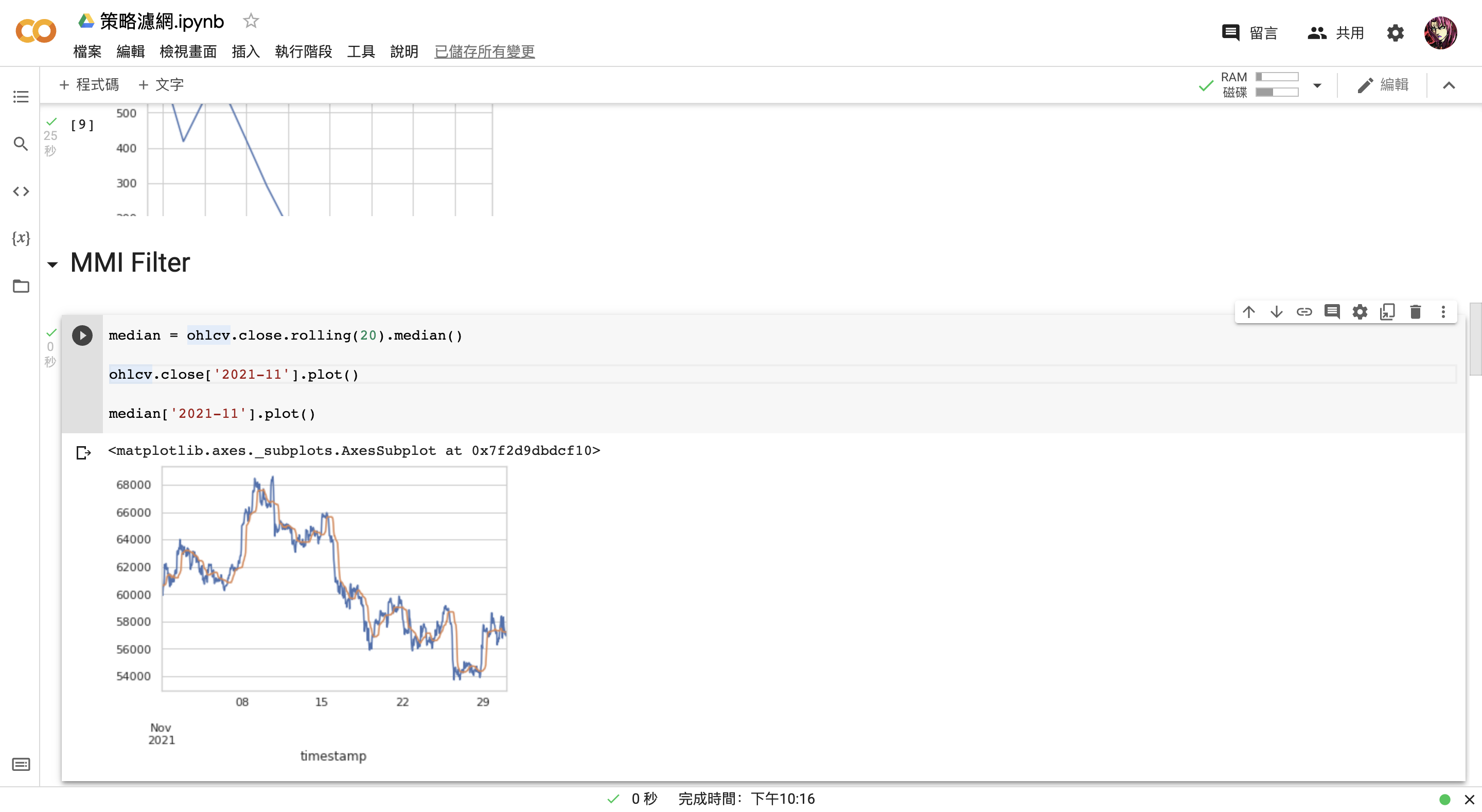median = ohlcv.close.rolling(20).median()

p1 = ohlcv.close > median

p2 = ohlcv.close.shift() > median

ohlcv.close['2021-11'].plot()

median['2021-11'].plot()


median = ohlcv.close.rolling(20).median()

p1 = ohlcv.close > median

p2 = ohlcv.close.shift() > median

mmi = (p1 & p2).astype(int).rolling(20).mean()

ohlcv.close['2021-11'].plot()

median['2021-11'].plot()

mmi['2021-11'].plot(secondary_y = True)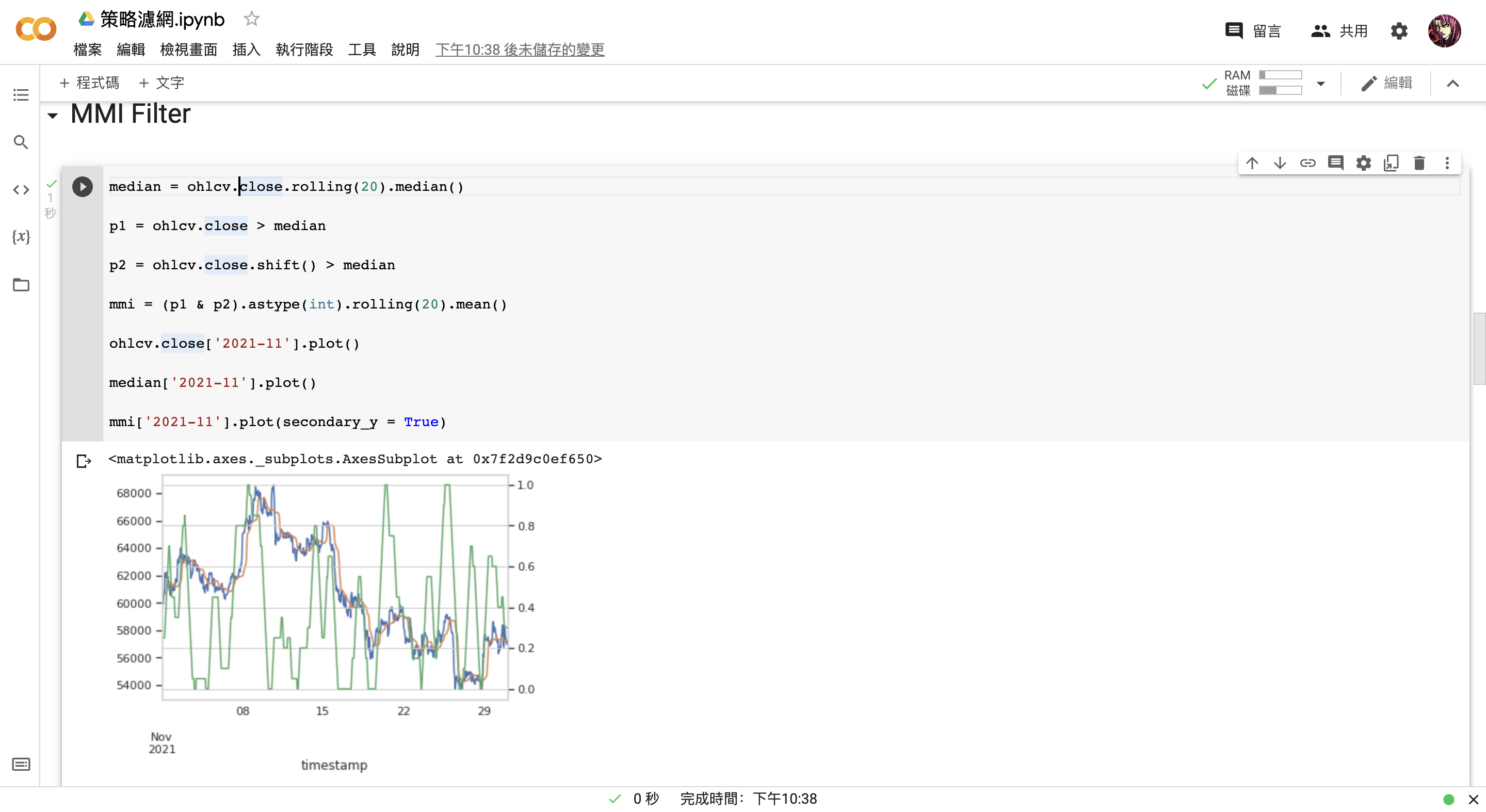entries = entries & (mmi > 0.5)


import numpy as np
from finlab_crypto import Strategy

@Strategy(sma1 = 20, sma2 = 60)
def mmi_sma_strategy(ohlcv):
close = ohlcv.close
sma20 = close.rolling(mmi_sma_strategy.sma1).mean()
sma60 = close.rolling(mmi_sma_strategy.sma2).mean()

entries = (sma20 > sma60) & (sma20.shift() < sma60.shift())
exits = (sma20 < sma60) & (sma20.shift() > sma60.shift())

figures = {
'overlaps': {
'sma20': sma20,
'sma60': sma60
}
}

median = ohlcv.close.rolling(20).median()

p1 = ohlcv.close > median

p2 = ohlcv.close.shift() > median

mmi = (p1 & p2).astype(int).rolling(20).mean()

entries = entries & (mmi > 0.5)

return entries, exits, figures

variables = {'sma1': np.arange(20, 100, 5), 'sma2': np.arange(20, 100, 5)}

portfolio = mmi_sma_strategy.backtest(ohlcv, variables=variables, freq='1h', plot=True)